Next: Rayleigh Scattering Up: Radiation and Scattering Previous: Antenna Arrays

# Thomson Scattering

When an electromagnetic wave is incident on a charged particle, the electric and magnetic components of the wave exert a Lorentz force on the particle, setting it into motion. Because the wave is periodic in time, so is the motion of the particle. Thus, the particle is accelerated and, consequently, emits radiation. More exactly, energy is absorbed from the incident wave by the particle, and re-emitted as electromagnetic radiation. Such a process is clearly equivalent to the scattering of the electromagnetic wave by the particle.

Consider a linearly polarized, monochromatic, plane wave incident on a particle of charge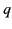. The electric component of the wave can be written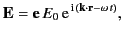(1273)

where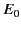is the peak amplitude of the electric field,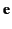is the polarization vector, and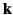is the wave vector (of course,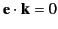). The particle is assumed to undergo small amplitude oscillations about an equilibrium position that coincides with the origin of the coordinate system. Furthermore, the particle's velocity is assumed to remain sub-relativistic, which enables us to neglect the magnetic component of the Lorentz force. The equation of motion of the charged particle is approximately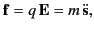(1274)

where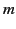is the mass of the particle,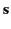is its displacement from the origin, and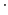denotes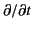. By analogy with Equation (1234), the time-averaged power radiated per unit solid angle by an accelerating, non-relativistic, charged particle is given by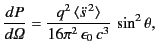(1275)

where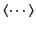denotes a time average. Here, we are effectively treating the oscillating particle as a short antenna. However,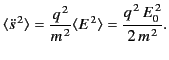(1276)

Hence, the scattered power per unit solid angle becomes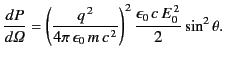(1277)

The time-averaged Poynting flux of the incident wave is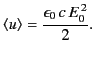(1278)

It is convenient to define the scattering cross-section as the equivalent area of the incident wavefront that delivers the same power as that re-radiated by the particle: that is,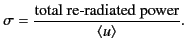(1279)

By analogy, the differential scattering cross-section is defined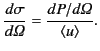(1280)

It follows from Equations (1279) and (1280) that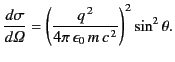(1281)

The total scattering cross-section is then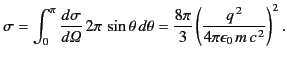(1282)

The quantity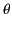, appearing in Equation (1283), is the angle subtended between the direction of acceleration of the particle, and the direction of the outgoing radiation (which is parallel to the unit vector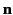). In the present case, the acceleration is due to the electric field, so it is parallel to the polarization vector. Thus,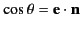.

Up to now, we have only considered the scattering of linearly polarized radiation by a charged particle. Let us now calculate the angular distribution of scattered radiation for the commonly occurring case of randomly polarized incident radiation. It is helpful to set up a right-handed coordinate system based on the three mutually orthogonal unit vectors,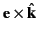, and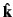, where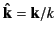. In terms of these unit vectors, we can write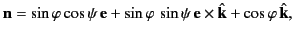(1283)

where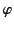is the angle subtended between the direction of the incident radiation and that of the scattered radiation, and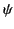is an angle that specifies the orientation of the polarization vector in the plane perpendicular to(assuming thatis known). It is easily seen that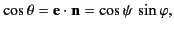(1284)

so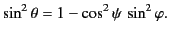(1285)

Averaging this result over all possible polarizations of the incident wave (i.e., over all possible values of the polarization angle), we obtain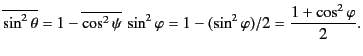(1286)

Thus, the differential scattering cross-section for unpolarized incident radiation [obtained by substituting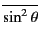for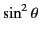in Eq. (1283)] is given by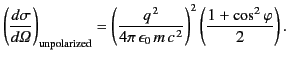(1287)

It is clear that the differential scattering cross-section is independent of the frequency of the incident wave, and is also symmetric with respect to forward and backward scattering. Moreover, the frequency of the scattered radiation is the same as that of the incident radiation. The total scattering cross-section is obtained by integrating over the entire solid angle of the polar angleand the azimuthal angle. Not surprisingly, the result is exactly the same as Equation (1284).

The classical scattering cross-section (1289) is modified by quantum effects when the energy of the incident photons,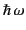, becomes comparable with the rest mass of the scattering particle,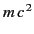. The scattering of a photon by a charged particle is called Compton scattering, and the quantum mechanical version of the Compton scattering cross-section is known as the Klein-Nishina cross-section. As the photon energy increases, and eventually becomes comparable with the rest mass energy of the particle, the Klein-Nishina formula predicts that forward scattering of photons becomes increasingly favored with respect to backward scattering. The Klein-Nishina cross-section does, in general, depend on the frequency of the incident photons. Furthermore, energy and momentum conservation demand a shift in the frequency of scattered photons with respect to that of the incident photons.

If the charged particle in question is an electron then Equation (1284) reduces to the well-known Thomson scattering cross-section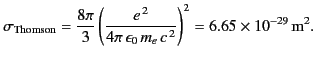(1288)

The quantity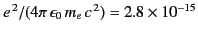m is called the classical electron radius (it is the radius of spherical shell of total chargewhose electrostatic energy equals the rest mass energy of the electron). Thus, when scattering radiation, the electron acts rather like a solid sphere whose radius is of order the classical electron radius.Next: Rayleigh Scattering Up: Radiation and Scattering Previous: Antenna Arrays
Richard Fitzpatrick 2014-06-27